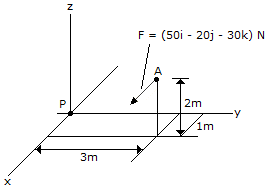# Engineering Mechanics - Force System Resultants - Discussion

### Discussion :: Force System Resultants - General Questions (Q.No.12)

12.Replace the force at A by an equivalent force and couple moment at P. Express the results on Cartesian vector form.

 [A]. F = (-50i+20j+30k) N, M = (50i-130j+170k) N-m [B]. F = (-50i+20j+30k) N, M = (-50i+60j+60k) N-m [C]. F = (50i-20j-30k) N, M = (-50i+130j-170k) N-m [D]. F = (50i-20j-30k) N, M = (50i-60j-60k) N-m

Explanation:

No answer description available for this question.

 Sagar said: (Jun 18, 2011) How to solve this ?